# The Paper LBO

Guide to Understanding the Paper LBO## The Paper LBO: Practice Tutorial Guide

Starting off, the interviewee typically receives a “prompt” – a short description containing a situational overview and certain financial data for a hypothetical company contemplating an LBO.

The interviewee will be given a pen and paper and 5–10 minutes to arrive at the implied IRR and other key metrics based exclusively on the information provided in the prompt.

For practically all private equity interviews, you will NOT be given a calculator — only pen and paper will be provided. In fact, it could even just be a verbal discussion with the interviewer.

So, you must practice doing mental math in your head until you are comfortable doing these shorthand calculations under pressure.

## Paper LBOs in Private Equity Interview

The paper LBO test is used by most private equity firms – and in some cases, even headhunters – to quickly vet a potential candidate and take place at fairly early stages of the PE interview process, i.e. the first round.

As candidates progress to subsequent rounds, private equity firms often ask interviewees to complete a far more detailed LBO modeling test, or perhaps even a take-home case study.

## How to Complete Paper LBOs?

Before we begin, the steps to complete a paper LBO test are outlined below.

• Step 1 → Input Transaction and Operational Assumptions
• Step 2 → Build “Sources & Uses” Table
• Step 3 → Project Financials
• Step 4 → Calculate Free Cash Flow (FCF)
• Step 5 → LBO Returns Analysis

## Illustrative Paper LBO Prompt

To get started, an example “prompt” for our modeling test tutorial can be found below.

###### Illustrative Prompt Example

JoeCo, a coffee company, has generated \$100mm in last twelve months (LTM) revenue and this figure is expected to increase by a growth rate of 10% annually into the foreseeable future.

JoeCo’s LTM EBITDA was \$50mm and its EBITDA margin should remain unchanged in the years ahead.

Based upon management guidance, JoeCo’s depreciation and amortization (D&A) and its capital expenditures (Capex) is expected to be 5.0% as a percentage of revenue, with no change in net working capital (NWC) and the effective tax rate fixed at 25%.

If a PE firm acquired JoeCo for 10.0x EBITDA and exited at the same multiple five years later, what is the implied internal rate of return (IRR) and multiple on invested capital (MOIC)?

For the financing of the LBO, assume the initial leverage ratio used to fund the purchase was 6.0x EBITDA and that the debt carries an interest rate of 8.0% with no required principal amortization until maturity, at which debt is fully paid down upon exit.

## Paper LBO Example – Excel Template

However, remember that in all likelihood, you will not receive an Excel sheet to work on during the interview.

Therefore, we recommend printing out the 1st sheet to solve this problem set, using pen and paper to familiarize yourself with the actual testing conditions.Submitting ...

## 1. Input Transaction and Operational Assumptions

The first step is to lay out the operational assumptions that were provided in the prompt and to calculate the total amount paid to purchase the target company as shown below: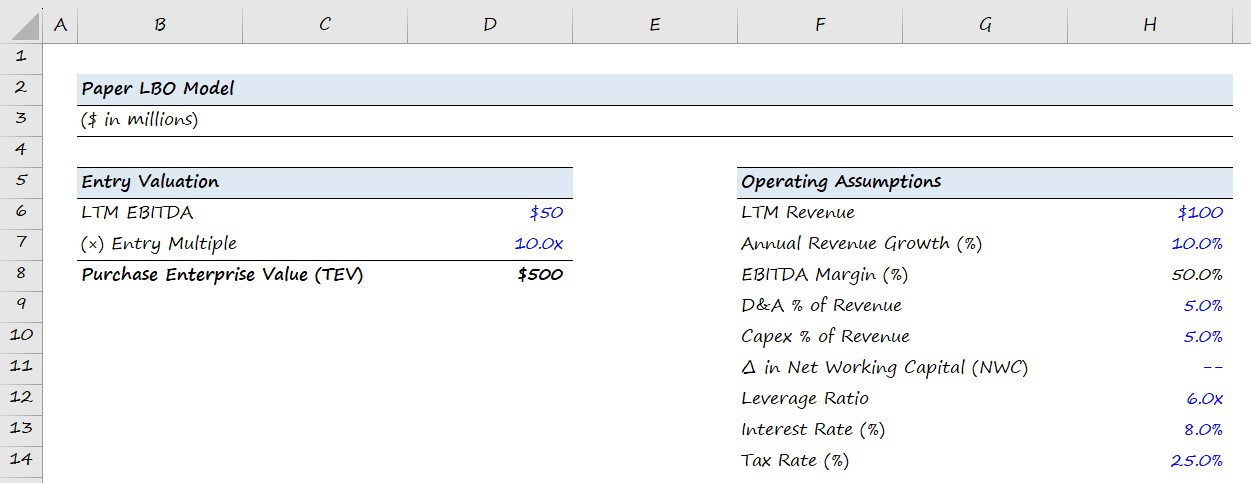Purchase Enterprise Value (TEV) = LTM EBITDA × Entry Multiple
EBITDA Margin (%) = LTM EBITDA ÷ LTM Revenue

## 2. Build Sources and Uses Table

Next, we will build out the Sources and Uses table, which will be a direct function of the transaction structure assumptions. In this particular example, the purchase multiple used was 10.0x EBITDA and the deal was funded using 6.0x leverage.

More specifically, the objective of this section is to figure out the exact cost of purchasing the company, and the amount of debt and equity financing that would be required to complete the acquisition.

The amount of debt used will be calculated as a multiple of LTM EBITDA, while the amount of equity contributed by the private equity investor will be the remaining amount required to “plug” the gap and make both sides of the table balance.

Ultimately, the main goal of an LBO model is to determine how much the firm’s equity investment has grown, and to do so – we need to first calculate the size of the initial equity check by the financial sponsor.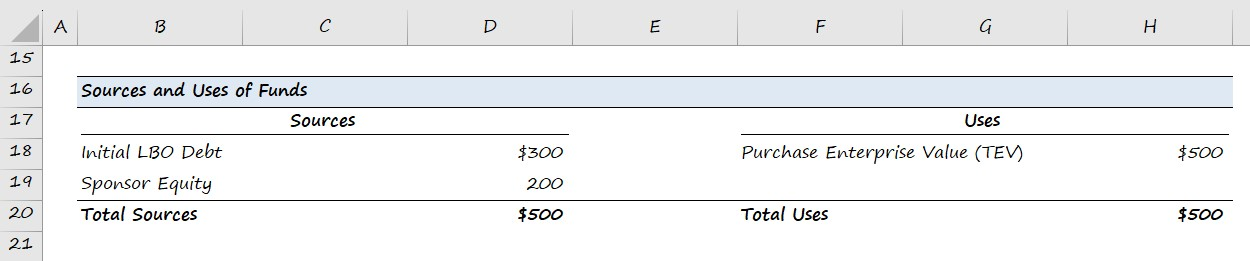Debt Financing = Leverage Multiple × LTM EBITDA
Sponsor Equity = Total Uses Debt Financing

In a real LBO model, the Uses of Funds Section will likely include transaction and financing fees, among other uses. In addition, other more complex concepts like management rollover will be reflected in both the sources and uses of funds.

However, these nuances are unlikely to show up here, so unless you were explicitly provided with additional data in the prompt, focus exclusively on the data provided.

## 3. Financial Forecast

We have completed filling out the Sources and Uses section of our model, so now we will project the financials of JoeCo down to net income (the “bottom line”).

The operational assumptions that will drive the projections were provided in the first step.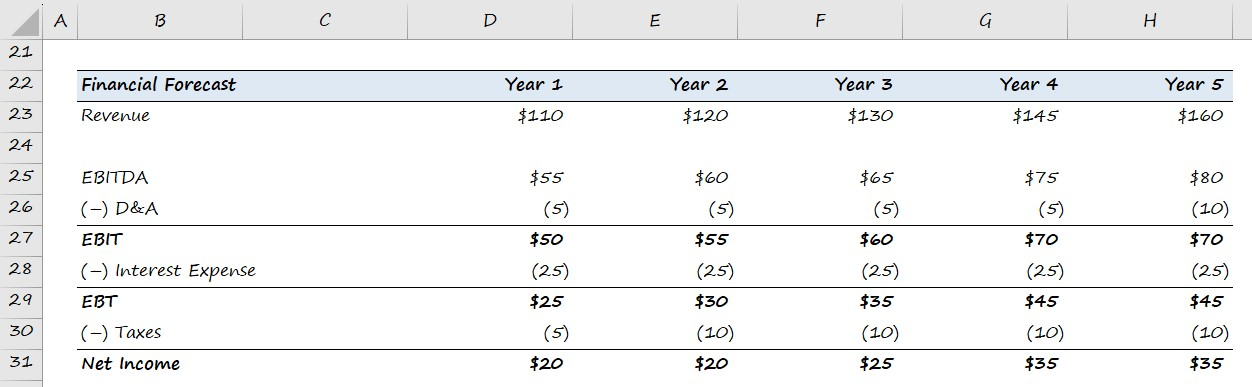As a quick side note, for interview purposes, it is reasonable (and expected) to round your calculations to the nearest whole number for convenience.

Revenue = Prior Period Revenue × (1 + Annual Revenue Growth Rate)
EBITDA = EBITDA Margin % × Current Period Revenue
D&A = D&A % of Revenue × Current Period Revenue
Interest Expense = Debt Financing Amount × Interest Rate %

## 4. Calculate Free Cash Flow (FCF)

Next, we will project JoeCo’s free cash flows (FCFs) throughout the five-year holding period.

The FCF generation ability of an LBO target will determine the amount of debt that can be paid down during the holding period – however, there will be no principal paydown assumed.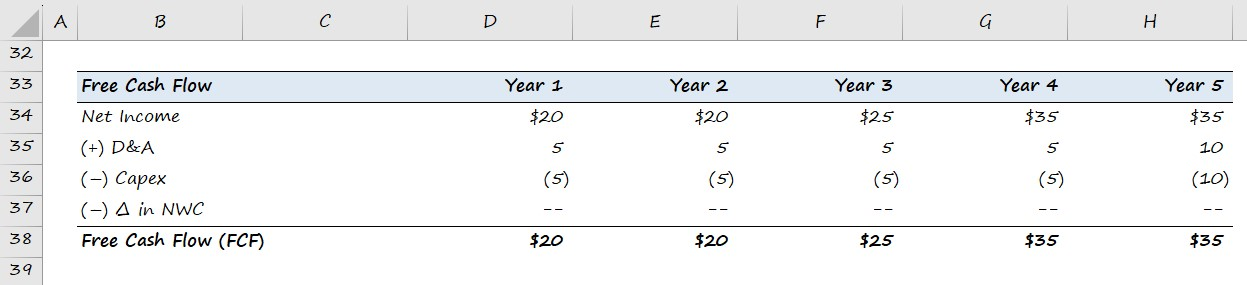Free Cash Flow (FCF) = Net Income + D&ACapexChange in NWC

## 5. LBO Returns Analysis (IRR and MOIC)

In the last step, we will assess the returns of the investment using the multiple on invested capital (MOIC), or “cash-on-cash return“, and the internal rate of return (IRR).

Earlier, the prompt stated that the PE firm exited the investment at the same multiple as the entry multiple (i.e. no “multiple expansion”).

Since you will likely not have access to a calculator, calculating the IRR requires some “back-of-the-envelope” math.

The standard investment holding period assumption is 5 years, so we recommend memorizing the IRRs based on the most common cash-on-cash-returns.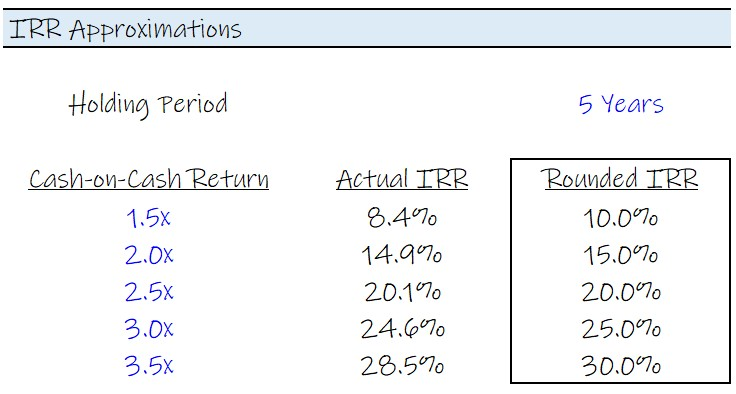###### The Rule of 72

Forgot your IRR approximations? No problem – in most cases, the return should be even easier to approximate under the Rule of 72, which estimates the time that it takes to double an investment as 72 divided by the rate of return.

For example, over a 5-year horizon, the approximate IRR required to double the investment is ~15%.

• Number of Years to Double = 72 ÷ 5 = ~15%

There’s also the lesser-known Rule of 115, which estimates the time it takes to triple an investment. Here, the formula takes 115 and divides it by the rate of return.

If you are facing difficulty estimating the IRR, there is a high likelihood that you may have made a mistake in a previous step.

For our paper LBO model, the implied MOIC is around 3.2x, which we calculated by dividing the exit equity value by the initial sponsor equity contribution.

Using either the table above or the Rule of 72 and 115, we can estimate the internal rate of return (IRR) on this LBO investment to be approximately ~26%.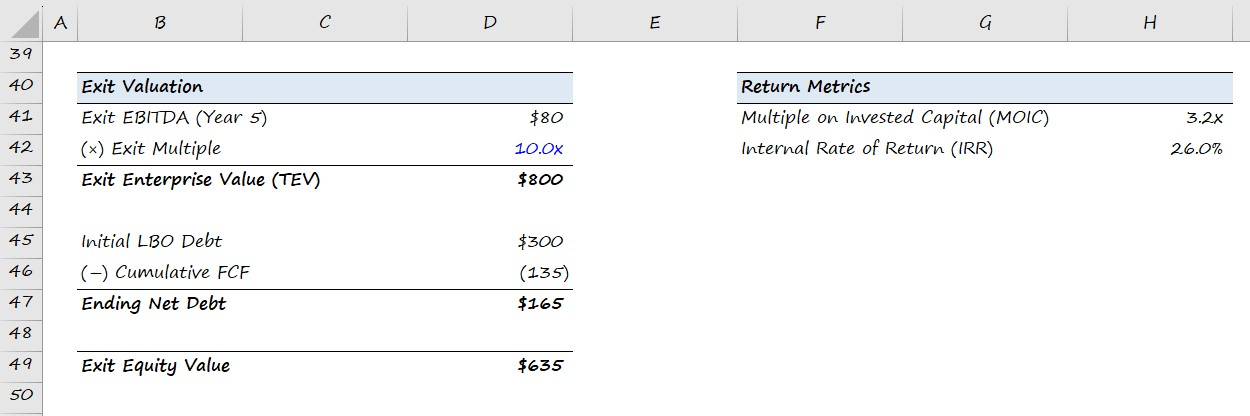Exit Enterprise Value = Exit Year EBITDA × Exit Multiple
Cumulative FCFs = Σ Free Cash Flows (FCFs)
Final Year Net Debt = Initial Debt AmountCumulative FCFs
Exit Equity Value = Exit Enterprise ValueEnding Year Net Debt
Multiple on Invested Capital (MOIC) = Exit Equity Value ÷ Initial Sponsor Equity
Internal Rate of Return (IRR) = MOIC ^ (1 ÷ t) – 1
Master LBO Modeling Our Advanced LBO Modeling course will teach you how to build a comprehensive LBO model and give you the confidence to ace the finance interview.Inline FeedbacksArt
February 9, 2022 3:40 pm

Wouldn’t you acquire the company on an NTM EBITDA number?

Last edited 1 year ago by Arthur DussiasFebruary 10, 2022 2:39 pm

Hi, Art,

Projected EBITDA is the norm for Comps analysis, to be sure, but LBOs usually work off of LTM EBITDA, that way the purchase multiple and leverage multiple can reference the same number, and leverage multiples almost always use historical numbers, because they are actual numbers.Joseph
June 4, 2022 10:53 am

I just completed this paper LBO – Thank you. In how long should this paper LBO need to have been completed?June 7, 2022 2:49 pm

Hi, Joseph,

The article says you should be able to arrive at the IRR in 5-10 minutes, so use that as a guide.

BBAllison Clark
March 16, 2023 12:47 pm

Does this get you to levered or unlevered FCF?March 16, 2023 3:38 pm